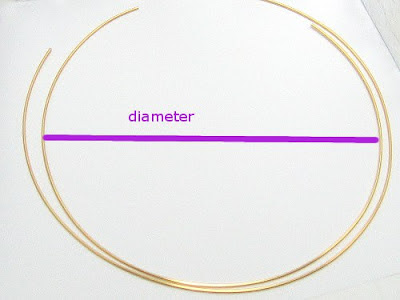## Sunday, March 11, 2012

### How to measure the length of wire?

Usually, it is not necessary to know the exact length of the while making.

When you want to know the length so that you will make your wireworking easier or not to waste your materials, you had better measure its length.

Because the materials we work on are not straight, we need to count the length of curve.

The ways to measure the length of wires:

• Use a cloth measurer
• Use mathematical formula: Circumference = diameter *  π (3.14)How to measure the length of wireThe mathematical circumference formula
The thumb rule:
A little bit "longer" is usually better than a little bit "shorter".